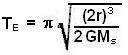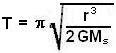## Wednesday, August 27, 2008

### Irodov Problem 1.203As in problem 1.202, the time period of a planet in an elliptical orbit with closest and farthest approach distances as R and r is given by,when the mass of the sun is Ms.

Suppose that the distance from earth to sun is r and that its a circle. The rotation period is given by,Now consider an extreme elliptical orbit that is almost like a straight line where R=0. Then the path will be almost as if the planet fell into the sun directly. The period of such a hypothetical orbit would be,However, the planet would only be able to complete half the orbit since it will fall into the sun and so the time taken to fall would be T/2.

So we have,